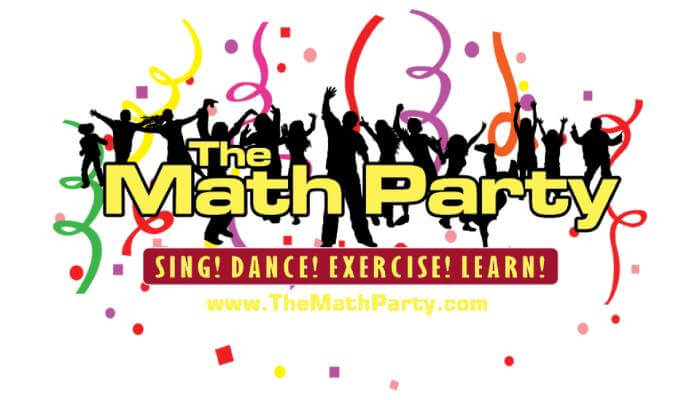TheMathParty## The Math Party Programs & Lessons#### Back To The Basics (Pre-K-2)

Count to 100

Count By 2’s

Count By 5’s

Count By 10’s

7 Days in a Week

12 Months in a Year

Ordinal Numbers

Telling Time I

Telling Time II

Sums from 6-10 (order)

Sums from 6-10 (mixed)

Sums 11-18 (order)

Sums 11-18 (mixed)

What Makes 5? What Makes 15?

What Makes 10? What Makes 20?

Subtraction Slide (0,1 Rules)

Subtract Form Numbers up to 5

Subtract 6-10 (order)

Subtract 6-10 (mixed)

Subtract 11-18 (order)

Subtract 11-18 (mixed)

Subtract From Numbers up to 18

Regrouping with Subtraction

Multiply X 1

Multiply X 2

Multiply X 3

Multiply X 4

Multiply X 5

Multiply X 6

Multiply X 7

Multiply X 8

Multiply X 9

Multiply X 10

Multiply X 11

Multiply X 12

#### State Test Prep (Grades 3-6)

The General Exam Overview

Place Value

Polygons

Divisions

Angles and Triangles

Ordering Decimals

Geometrical Figures

Measurements

#### Number & Operations l (Grades 4-8)

Place Value (Whole Numbers)

Ordering and Comparing Decimals

Multiplying Decimals

Converting Decimals to Percents/Percents to Decimals

Converting Decimals to Fractions

Converting Fractions to Decimals

Factors, Multiples, Composite Numbers, Prime Numbers

Rounding Whole Numbers

Division

#### Number & Operations ll (Grades 4-8)

Equivalent Fractions

Reducing Fractions to Simplest Form

Comparing and Ordering Fractions

Subtraction of Unlike Fractions

Multiplying Fractions

Dividing Fractions

Converting Improper Fractions  to Mixed Numbers

Converting Mixed Numbers to Improper Fractions

#### Number, Operations & Algebra (Grades 4-8)

Discount Price

Sales Tax

Sum, Difference, Products, Quotients

Order of Operations

Subtracting Integers

Multiplying Integers

Dividing Integers

Associative, Commutative, Identity Properties

Exponents

#### Geometry, Data Analysis & Probability (Grades 4-8)

Polygons

Transformations

Line, Ray, Line Segment, Plane, Point

Geometric Figures

Solid Figures

Classifying Triangles

Line Relationships

Graphing Integers on a Coordinate Plane

Graphs

Probability

Measures of Central Tendency

Degrees in Angles

Metric Units of Mass

Metric Units Units of Length

Metric Units of Capacity

Customary Units of Length

Customary Units of Capacity

Customary Units of Weight

Measures of Time

Area, Perimeter, Volume, CircumferenceLetter A

Letter B

Letter C

Letter D

Letter E

Letter F

Letter G

Letter H

Letter I

Letter J

Letter K

Letter L

Letter M

Letter N

Letter O

Letter P

Letter Q

Letter R

Letter S

Letter T

Letter U

Letter V

Letter W

Letter X

Letter Y

Letter Z

#### Phonics/Fitness/Fundamentals (Pre-K-2)

ABC Song

Letters and Sounds

Letters/Sounds/Words

Short Vowels

Long Vowels

W and Y as Vowels

Consonants

Segments and Blends

Syllables

Prefixes

Suffixes

Compound Words

Rhyming

Consonant Blends with L

Consonant Blends with R

Consonant Blends with S

Consonant Digraphs

Vowel Digraphs

Vowel Diphthongs